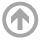Electrical and Computer Engineering Department

# EE432: Digital Signal Processing

Course Description – EE432 Digital Signal Processing (3-2-4):  A follow-on to EE322: Signals and Systems, this course further explores the creation (A/D) and processing of discrete signals and systems, which are analyzed both in the time and in frequency domains. The Discrete Time Fourier Transform and Z-Transform are introduced. System properties such as stability, linearity and time-invariance are studied. Focusing on linear time-invariant (LTI) systems, linear constant coefficient difference equations (LCCDE) are used to relate the time-domain input-output relationship of a system to the system’s frequency response. Digital filters, both FIR and IIR, are designed to meet specifications and applied to discrete inputs such as voice, music and biomedical signals.

Course Objectives – Students shall be able to:

1. Analyze the frequency content of a digital signal and the frequency response of a digital system using the Discrete-Time Fourier Transfer (DTFT) and the z-Transform (ZT). Explain the relationship between the DTFT and the Discrete Fourier Transform (DFT).
2. Describe the Analog-to-Digital (A/D) and Digital-to-Analog (D/A) conversion processes, including the relationship between sampling and aliasing, and give the Nyquist criterion for sampling a bandlimited analog signal.
3. Demonstrate an understanding of the various ways to characterize and implement digital filters, both FIR and IIR (Linear Constant Coefficient Difference Equations (LCCDE), system function, impulse response, poles/zeros, block diagrams).
4. Use MATLAB to determine and analyze the frequency content of digital signals, the frequency response of digital systems, and system outputs for given system inputs such as voice, music and biomedical signals.
5. In filter design, discuss the implications of pole/zero placement, the difference between the choice of FIR or IIR filters, and how the choice of different windows (length and type) affects system frequency response. Use MATLAB to design filters to given specifications.